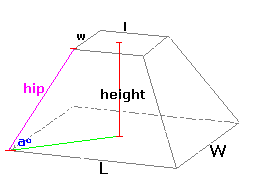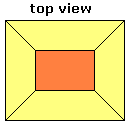Spike's Calculators

# Truncated Rectangle Pyramid Volume and Surface Area

Calculate the volume and surface area of a truncated rectangular pyramid.This calculator works for any measurement unit. For example, if you calculate inches, inches will have to be used in all the length fields, and the result of the areas will be square inches; the volume results in cubic inches and length measurements in the starting unit, inches.

### Truncated Rectangular Pyramid

Upper Base Length #
Upper Base Width #
Lower Base Length #
Lower Base Width #
Height #
Decimal Precision #

#### Results:

 Pyramid Volume #³ Base Area #² Top Area #² Lateral Area #² Surface Area #² Hip Length # Angle a ° Surface to Volume Ratio sa:vol

#### Calculation

• the length of the upper base
• the width of the upper base
• the length of the lower base
• the width of the lower base
• the height of the truncated pyramid
• decimal precision, number of digits after the decimal point

#### Results

• the pyramid volume (unit cubed)
• the base (bottom) area of the pyramid (unit squared)
• the top area of the pyramid (unit squared)
• the lateral area (unit squared)
• the surface area unit squared)
• the hip length (outside diagonal) (starting unit)
• angle a (degrees)
• surface area to volume ratio (decimal)
##### Formula
```V = H/6(Lw+Wl+2(lw+LW)
B = LW+lw
LA = 2((L+l)/2e+(W+w)/2f))
e = (W-w)²+H²
f = (L-l)²+H²
SA = LA+BA
D =√L²+W²
HL = √(D/2)²+H²
a = sin-1(H/(D/2))
s/v = SA/V
where V is the volume of the pyramid
L the length of the base
W the width of the base
H the height of the pyramid
BA the area of the base
LA the lateral suface area
SA the surface area of the pyramid
D the base diagonal length
HL the length of the hip (outside edge)
a the angle of the hip
s/v is the surface area to volume ratio
```